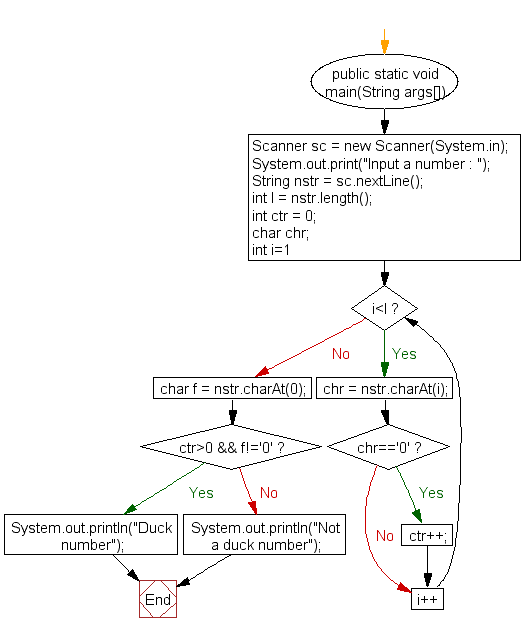﻿ Java exercises: Check whether a number is a Duck Number or not - w3resourceJava Exercises: Check whether a number is a Duck Number or not

Java Numbers: Exercise-15 with Solution

Write a Java program to check whether a number is a Duck Number or not.

Note: A Duck number is a number which has zeroes present in it, but there should be no zero present in the beginning of the number. For example 3210, 7056, 8430709 are all duck numbers whereas 08237, 04309 are not.

Test Data
Input a number : 3210

Pictorial Presentation:Sample Solution:

Java Code:

``````import java.util.Scanner;

public class Example15  {

public static void main(String args[])
{
Scanner sc = new Scanner(System.in);
System.out.print("Input a number : ");
String nstr = sc.nextLine();

int l = nstr.length();
int ctr = 0;
char chr;

for(int i=1;i<l;i++)
{
chr = nstr.charAt(i);
if(chr=='0')
ctr++;
}

char f = nstr.charAt(0);

if(ctr>0 && f!='0')
System.out.println("Duck number");
else
System.out.println("Not a duck number");
}
}
```
```

Sample Output:

```Input a number : 3210
Duck number
```

Flowchart:Java Code Editor: